Download Presentation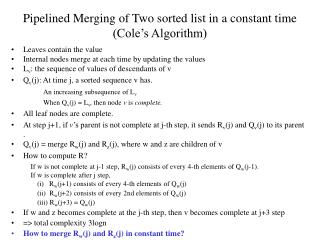Pipelined Merging of Two sorted list in a constant time (Cole’s Algorithm)

# Pipelined Merging of Two sorted list in a constant time (Cole’s Algorithm) - PowerPoint PPT Presentation

Pipelined Merging of Two sorted list in a constant time (Cole’s Algorithm). Leaves contain the value Internal nodes merge at each time by updating the values L v : the sequence of values of descendants of v Q v (j): At time j, a sorted sequence v has. An increasing subsequence of L vI am the owner, or an agent authorized to act on behalf of the owner, of the copyrighted work described.
Download Presentation## Pipelined Merging of Two sorted list in a constant time (Cole’s Algorithm)

An Image/Link below is provided (as is) to download presentation

Download Policy: Content on the Website is provided to you AS IS for your information and personal use and may not be sold / licensed / shared on other websites without getting consent from its author.While downloading, if for some reason you are not able to download a presentation, the publisher may have deleted the file from their server.

- - - - - - - - - - - - - - - - - - - - - - - - - - E N D - - - - - - - - - - - - - - - - - - - - - - - - - -
Presentation Transcript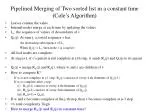Pipelined Merging of Two sorted list in a constant time(Cole’s Algorithm)
• Leaves contain the value
• Internal nodes merge at each time by updating the values
• Lv: the sequence of values of descendants of v
• Qv(j): At time j, a sorted sequence v has.

An increasing subsequence of Lv

When Qv(j) = Lv, then node v is complete.

• All leaf nodes are complete.
• At step j+1, if v’s parent is not complete at j-th step, it sends Rv(j) and Qv(j) to its parent.
• Qv(j) = merge Rw(j) and Rz(j), where w and z are children of v
• How to compute R?

If w is not complete at j-1 step, Rw(j) consists of every 4-th elements of Qw(j-1).

If w is complete after j step,

(i) Rw(j+1) consists of every 4-th elements of Qw(j)

(ii) Rw(j+2) consists of every 2nd elements of Qw(j)

(iii) Rw(j+3) = Qw(j)

• If w and z becomes complete at the j-th step, then v becomes complete at j+3 step
• => total complexity 3logn
• How to merge Rw(j) and Rz(j) in constant time?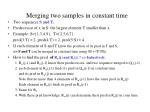Merging two samples in constant time
• Two sequences S and T.
• Predecessor of x in S: the largest element T smaller than x.
• Example: S={1,3,4,9}, T={2,5,6,7}

pred(3:T) = 2, pred(4:T) = 2, pred(5:S) = 4.

• If each element of S and T know the position of its pred in T and S,

=> S and T can be merged in constant time using |S| + |T| PEs.

• How to find the pred of Rw(j) and Rz(j) ? => Inductively.

1.Rw(j-1) and Rz(j-1) know their predecessors, and two sequence merged to Qv(j-1) .

2. each element in Rw(j-1) finds its pred in Qw(j-1) in constant time

and its pred in Rw(j) in constant time.

Note that no more than 4 elements of Rw(j-1) have the same pred in Rw(j)

Each element in Rw(j) finds its pred in Rw(j-1)

3. Same for Rz.

4. With these pred knowledge, Rw(j) can determine their pred in Rz(j) in cons time.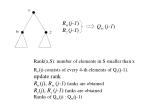v

Rw(j-1)

Rz (j-1)

Qw (j-1)

w

z

Rank(x,S): number of elements in S smaller than x

Rv(j) consists of every 4-th elements of Qv(j-1).

update rank

Rw(j), Rw (j-1) ranks are obtained

Rz(j), Rz (j-1) ranks are obtained

Ranks of Qw(j) : Qw(j-1)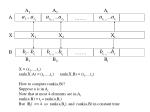A1

A2

Ai

A

…….

X

X1

X2

Xi

B

…….

B1

B2

Bi

X = (x1,...,xs)

rank(X:A) = (r1,...,rs) rank(X,B) = (t1,...,ts)

How to compute rank(a,B)?

Suppose a is in Ai

Note that at most 4 elements are in Ai

rank(a:B) = ti + rank(a,Bi)

But |Bi| <= 4 so rank(a,Bi), and rank(a,B) in constant time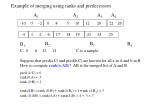Example of merging using ranks and predecessors

A1

A2

A3

A4

-10 -5 -2 0 4 5 10 12 20 22 26

-4 1 2 6 17 18 19 21 24 25

B2

B3

B4

B1

C: 0 6 12 21 C is a sample

Suppose that pred(a,C) and pred(b,C) are known for all a in A and b in B

How to compute rank(x:AB)? AB is the merged list of A and B

pred (4:C) = 0

rank(0,A) = 3

rank (0:B) = 1

rank(4,B) = rank (0:B) + rank(4,B2) = 1+rank (4,B2) = 3

rank (4:AB) = rank(4,A) + rank(4,B) = 4 + 3 = 7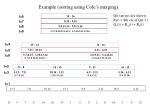Example (sorting using Cole’s merging)

Q(t) arrays are shown

R(t) = 4th ele of Q(t-1)

Qv(t) = Rw(t) + Rz(t)

t=6

15 : 16

t=7

8,15 : 9,16

t=8

5,8,13,15 : 3,9,11,16

t=9

1,5,7,8,10,13,14,15 : 2,3,4,6,9,11,12,16

t=4

15 : 14 11 : 16

7,15 : 10,14 6,11 : 4,16

t=5

5,7,8,15 : 1,10,13,14 2,6,9,11 : 3,4,12,16

(1,5,7,8,10,13,14,15) (2,3,4,6,9,11,12,16)

t=6

t=2

15 : 8 14 : 13 9 : 11 16 : 12

t=3

5,15 : 7,8 10,14 : 1,13 6,9 : 2,11 3,16 : 4,12

(5 7 8 15) ( 1 10 13 14) (2 6 9 11) (3 4 12 16)

t=1

15:5 7,8 10,14 13,1 6,9 2,11 16,3 4,12

15 5 7 8 14 10 13 1 6 9 2 11 16 3 4 12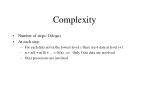Complexity
• Number of steps: O(logn)
• At each step:
• For each data not in the lowest level i, there are 4 data at level i+1
• n + n/4 + n/16 + ... = O(n) => Only O(n) data are involved
• O(n) processors are involved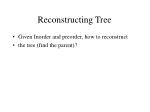Reconstructing Tree
• Given Inorder and preorder, how to reconstruct
• the tree (find the parent)?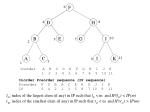6

F

4

D

H

8

B

E

G

J

10

7

5

2

A

C

I

K

11

9

3

1

Inorder A B C D E F G H I J K

1 2 3 4 5 6 7 8 9 10 11

Inorder Preorder sequence (IP sequence)

Preorder F D B A C E H G J I K

IP 6 4 2 1 3 5 8 7 10 9 11

lm: index of the largest elem (if any) in IP such that lm < m and IP(lm) < IP(m)

rm: index of the smallest elem (if any) in IP such that rm < m and IP(rm) > IP(m)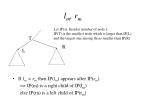Let IP(x): Inorder number of node x.

IP(T) is the smallest node which is larger than IP(L)

and the largest one among those smaller than IP(R)

T

R

L

lm, rm
• If lm > rm then IP(lm) appears after IP(rm)

=> IP(m) is a right child of IP(lm)

else IP(m) is a left child of IP(rm)Example

Inorder A B C D E F G H I J K

m 1 2 3 4 5 6 7 8 9 10 11

IP(m) 6 4 2 1 3 5 8 7 10 9 11

F D B A C E H G J I K

IP(lm) -- -- -- -- 2 4 6 6 8 8 1

node B D F F H H J

lm 3 2 1 1 7 7 9

IP(rm) -- 6 4 2 4 6 - 8 - 10 -

node (F) D B D F - H - J -

rm 1 2 3 2 1 - 7 - 9 -

f(m) = m, y(m) = IP(m)

* defined:

i*j = i provided IP(i) > IP(j)

= j otherwise

D(m) gives lm

Operation *: max

f(m) = y(m) = IP(m)

D(m) gives IP(lm)

Operation *: min

f(m) = IP(m), y(m) = -IP(m)

D(m) gives IP(rm)